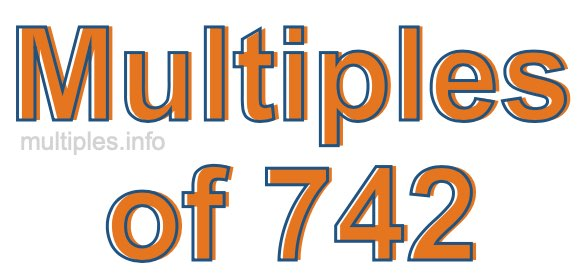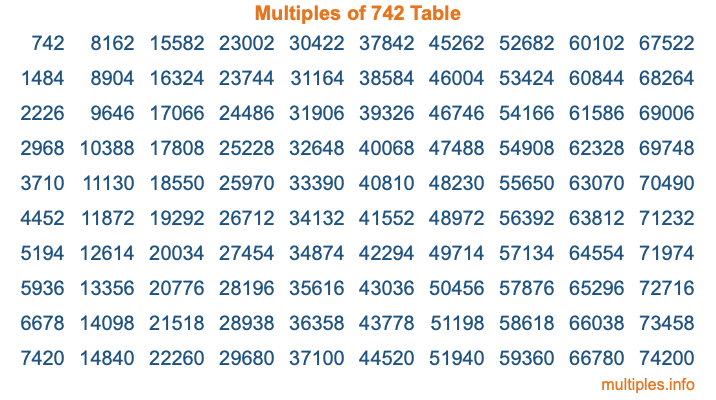Multiples of 742Welcome to the Multiples of 742 page. Here we will first teach you everything you will ever need to know about the multiples of 742, and then give you a study guide summary of everything we taught you to make sure you remember it all. Use this page to look up facts and learn information about the multiples of 742. This page will make you a multiples of seven hundred forty-two expert!

Definition of Multiples of 742
Multiples of 742 are all the numbers that when divided by 742 equal an integer. Each of the multiples of 742 are called a multiple. A multiple of 742 is created by multiplying 742 by an integer.

Therefore, to create a list of multiples of 742, you start with 1 multiplied by 742, then 2 multiplied by 742, then 3 multiplied by 742, and so on for as long as you want. Thus, the list of the first five multiples of 742 is 742, 1484, 2226, 2968, and 3710. To see a larger list of multiples of 742, see the printable image of Multiples of 742 further down on this page. We also have a category where you can choose any nth multiple of 742.

Multiples of 742 Checker
The Multiples of 742 Checker below checks to see if any number of your choice is a multiple of 742. In other words, it checks to see if there is any number (integer) that when multiplied by 742 will equal your number. To do that, we divide your number by 742. If the the quotient is an integer, then your number is a multiple of 742.

Is  a multiple of 742?

Least Common Multiple of 742 and ...
A Least Common Multiple (LCM) is the lowest multiple that two or more numbers have in common. This is also called the smallest common multiple or lowest common multiple and is useful to know when you are adding our subtracting fractions. Enter one or more numbers below (742 is already entered) to find the LCM.

Check out our LCM Calculator if you need more details about the Least Common Multiple or if you need the LCM for different numbers for adding and subtraction fractions.

nth Multiple of 742
As we stated above, 742 is the first multiple of 742, 1484 is the second multiple of 742, 2226 is the third multiple of 742, and so on. Enter a number below to find the nth multiple of 742.

th multiple of 742

Multiples of 742 vs Factors of 742
742 is a multiple of 742 and a factor of 742, but that is where the similarities end. All postive multiples of 742 are 742 or greater than 742. All positive factors of 742 are 742 or less than 742.

Below is the beginning list of multiples of 742 and the factors of 742 so you can compare:

Multiples of 742: 742, 1484, 2226, 2968, 3710, etc.

Factors of 742: 1, 2, 7, 14, 53, 106, 371, 742

As you can see, the multiples of 742 are all the numbers that you can divide by 742 to get a whole number. The factors of 742, on the other hand, are all the whole numbers that you can multiply by another whole number to get 742.

It's also interesting to note that if a number (x) is a factor of 742, then 742 will also be a multiple of that number (x).

Multiples of 742 vs Divisors of 742
The divisors of 742 are all the integers that 742 can be divided by evenly. Below is a list of the divisors of 742.

Divisors of 742: 1, 2, 7, 14, 53, 106, 371, 742

The interesting thing to note here is that if you take any multiple of 742 and divide it by a divisor of 742, you will see that the quotient is an integer.

Multiples of 742 Table
Below is an image of the first 100 multiples of 742 in a table. The table is in chronological order, column by column. The first column has the first ten multiples of 742, the second column has the next ten multiples of 742, and so on.The Multiples of 742 Table is also referred to as the 742 Times Table or Times Table of 742. You are welcome to print out our table for your studies.

Negative Multiples of 742
Although not often discussed or needed in math, it is worth mentioning that you can make a list of negative multiples of 742 by multiplying 742 by -1, then by -2, then by -3, and so on, to get the following list of negative multiples of 742:

-742, -1484, -2226, -2968, -3710, etc.

Multiples of 742 Summary
Below is a summary of important Multiples of 742 facts that we have discussed on this page. To retain the knowledge on this page, we recommend that you read through the summary and explain to yourself or a study partner why they hold true.

There are an infinite number of multiples of 742.

A multiple of 742 divided by 742 will equal a whole number.

742 divided by a factor of 742 equals a divisor of 742.

The nth multiple of 742 is n times 742.

The largest factor of 742 is equal to the first positive multiple of 742.

742 is a multiple of every factor of 742.

742 is a multiple of 742.

A multiple of 742 divided by a divisor of 742 equals an integer.

742 divided by a divisor of 742 equals a factor of 742.

Any integer times 742 will equal a multiple of 742.

Multiples of a Number
Here you can get the multiples of another number, all with the same attention to detail as we did for multiples of 742 on this page.

Multiples of
Multiples of 743
Did you find our page about multiples of seven hundred forty-two educational? Do you want more knowledge? Check out the multiples of the next number on our list!